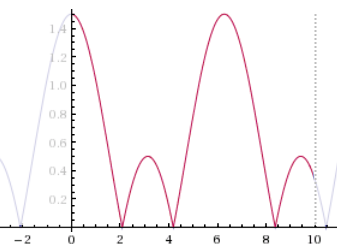# Local Extrema CountThe graph at right depicts the function $\color{darkred}{f(x)} = |\cos x + 0.5|$ in the interval $\color{darkred}0 \leq \color{darkred}x \leq \color{darkred}{10}$.

How many local extrema does the function $f(x)$ have if its domain is restricted to ${\color{darkred}0 \leq \color{darkred}x \leq \color{darkred}{10}}?$

×